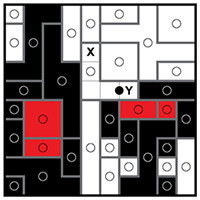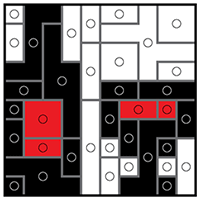﻿ Sym-a-Pix решение
 ВходРегистрация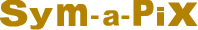# Sym-a-Pix решение

Sym-a-Pix puzzles consist of a grid with dots in various places, where each dot is the center of a rotationally symmetric block and each block contains one dot. In addition, each block is painted according to the color of the dot in its center. When the puzzle is completed, a hidden pixel-art picture is revealed. Sym-a-Pix puzzles come in two levels, Basic Logic and Advanced Logic. Basic Logic Sym-a-Pix require easy logic techniques and are straightforward to solve while Advanced Logic Sym-a-Pix contain more advanced situations making these puzzles very challenging and rewarding to solve.

# Basic Logic Sym-a-Pix step by step solution

Basic Logic Sym-a-Pix puzzles are solved by drawing walls around the dots according to the rules until the rotationally symmetric blocks are formed around all dots. The best way to learn how to solve Basic Logic Sym-a-Pix is to see a puzzle solved step by step from beginning to end.

## Step 1

The first step in solving Basic Logic Sym-a-Pix is to draw walls between adjacent squares containing dots or fractions of dots as shown below. Since a square containing a dot or a fraction of a dot must belong to the block surrounding that dot, whenever two adjacent squares contain different dots or fractions of different dots they must be separated by a wall. After drawing the walls we can paint the completed blocks in black according to the color of the dots.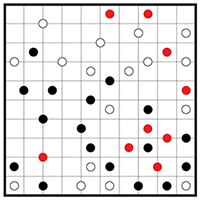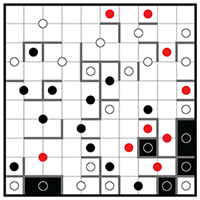## Step 2

The next step is to draw walls around the dots according to the rule of rotational symmetry and paint the completed blocks in according to the color of the dots. Note that the puzzle borders also serve as walls.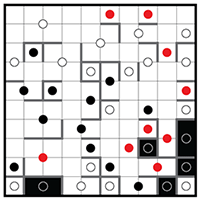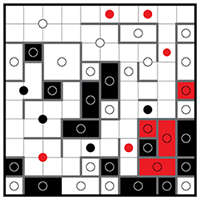## Step 3

We now see three areas marked with X in which the squares must belong to a specific dot. We name such situations as captured squares. Since these captured squares must have rotationally symmetric squares on the other side of the dot we can draw additional walls and paint the completed blocks according to the color of the dots.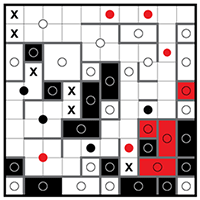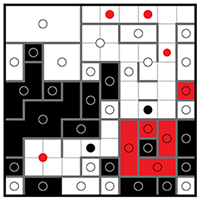## Step 4

The previous step creates two new areas with captured squares marked with X which are solved in the same way as above, as well as two areas marked with Y which are solved by drawing walls according to the rule of rotational symmetry.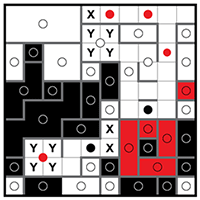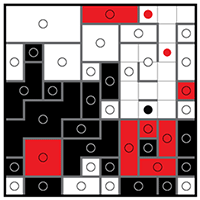## Step 5

The next step is to draw the last wall around the square with the X according to the rule of rotational symmetry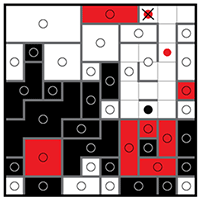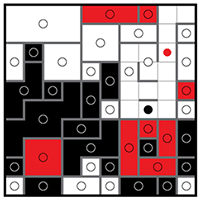## Step 6

And again another area with captured squares is created which is solved according to the rule of rotational symmetry. The necessary walls are drawn and the completed block is painted in red according to the color of the dot.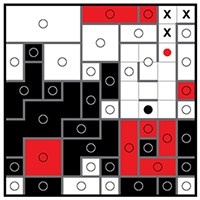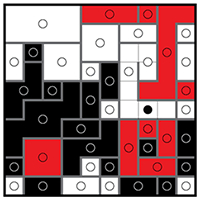## Step 7

Using the same techniques as above, we can now complete the puzzle. The Basic Logic Sym-a-Pix puzzle is now complete, revealing the picture of a tractor.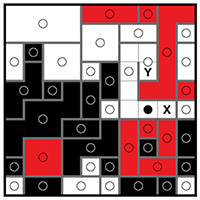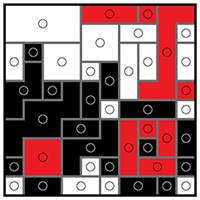# Advanced Logic Sym-a-Pix step by step solution

Unlike Basic Logic, Advanced Logic Sym-a-Pix puzzles contain additional situations where it is not clear to which dot a square belongs. The best way to learn how to solve Advanced Logic Sym-a-Pix is to see a puzzle solved step by step from beginning to end.

## Step 1

The first step in solving Advanced Logic Sym-a-Pix is to draw walls between adjacent squares containing dots or fractions of dots as shown below. Since a square containing a dot or a fraction of a dot must belong to the block surrounding that dot, whenever two adjacent squares contain different dots or fractions of different dots they must be separated by a wall. After drawing the walls we can paint the completed blocks according to the color of the dots.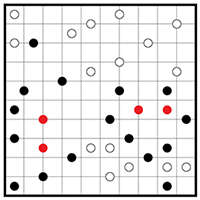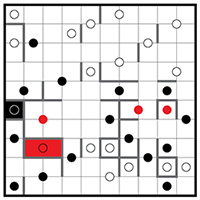## Step 2

The next step is to draw walls around the dots according to the rule of rotational symmetry and paint the completed blocks according to the color of the dots. Note that the puzzle borders also serve as walls.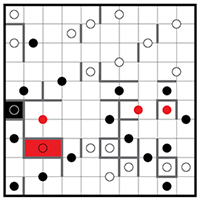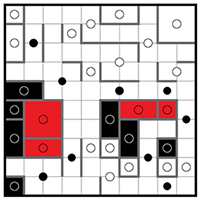## Step 3

We now see two areas marked with X in which the squares must belong to a specific dot. We name such situations as captured squares. Since these captured squares must have rotationally symmetric squares on the other side of the dot we can draw additional walls and paint the completed blocks according to the color of the dots.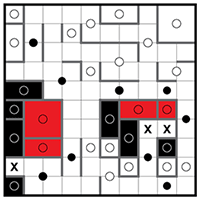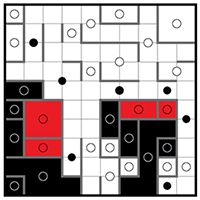## Step 4

The previous step creates one new area with a captured square marked with X which is solved in the same way as above, as well as three areas marked with Y which are solved by drawing walls according to the rule of rotational symmetry.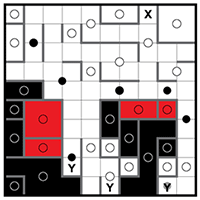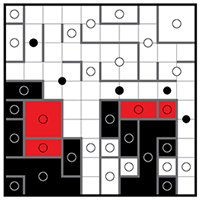## Step 5

This step is solved using the same captured squares and rotational symmetry techniques as in the previous step.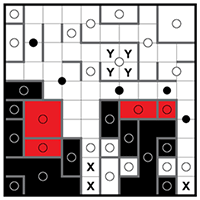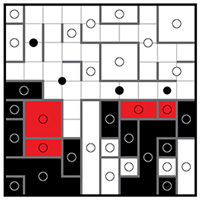## Step 6

We now come across situations requiring advanced logic. The square with the question mark on the left side of the puzzle can belong to one of two dots. However, it cannot belong to the lower of these two dots because the rotationally symmetric square already contains a dot. Therefore the square with the question mark on the left must belong to the upper dot of the two. Similarly, the square with the question mark on the right side of the puzzle must belong to the dot on its upper left. All rotationally symmetric walls can now be drawn and the completed blocks painted according to the color of the dots.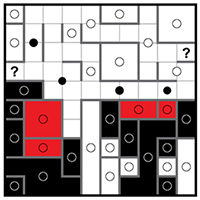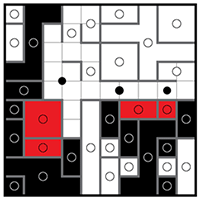## Step 7

The previous advanced logic step creates one new area with captured squares marked with X which is solved in the same way as before, as well as three areas marked with Y which are solved by drawing walls according to the rule of rotational symmetry.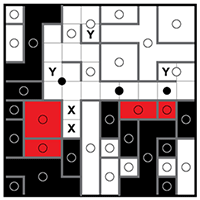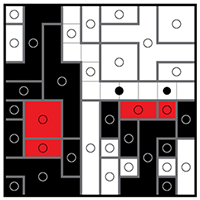## Step 8

And again another two areas with captured squares are created which are solved according to the rule of rotational symmetry. The necessary walls are drawn and the completed blocks are painted according to the color of the dots.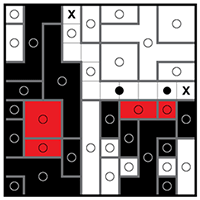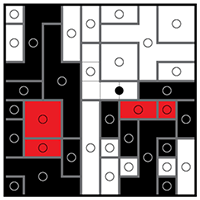## Step 9

Using the same techniques as above, we can now complete the puzzle. The Advanced Logic Sym-a-Pix puzzle is now complete, revealing the picture of a wine bottle and a glass.